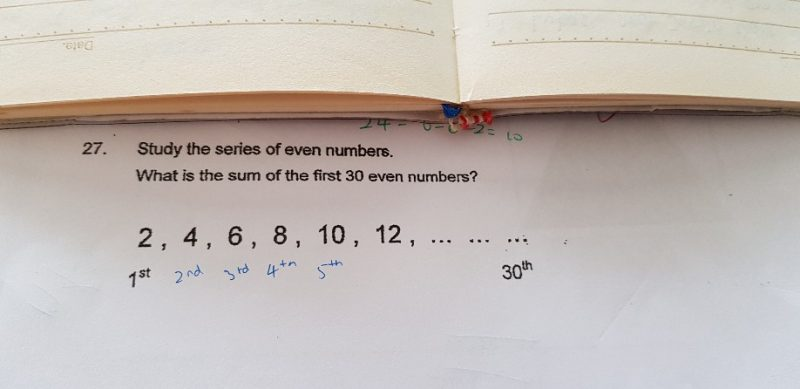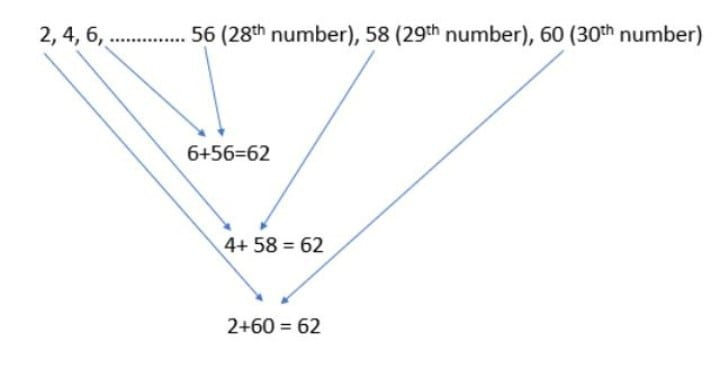# QuestionThere are a total of 15 sets of these “62” numbers.

So the total sum of the numbers = 15 * 62 = 930 (ans)

0 Replies 0 Likes

30th no => 30 x 2 = 60

Sum of first 30th even numbers will be,

(60 + 2) x 30/2 = 930

0 Replies 0 Likes